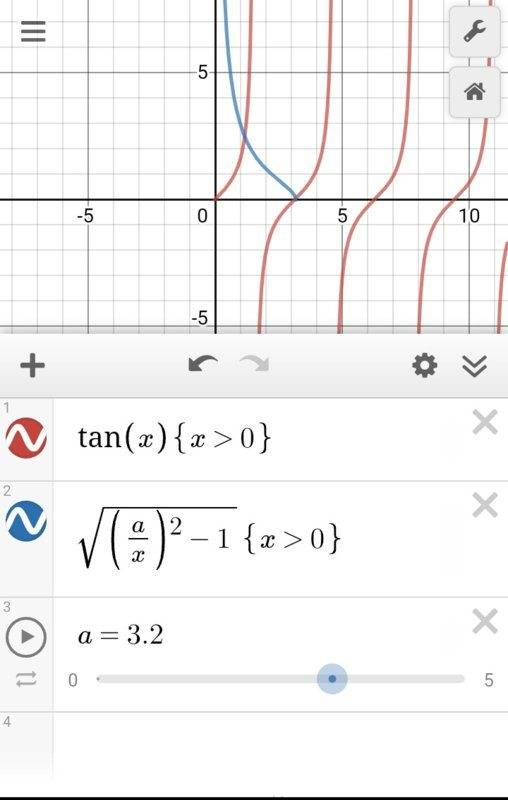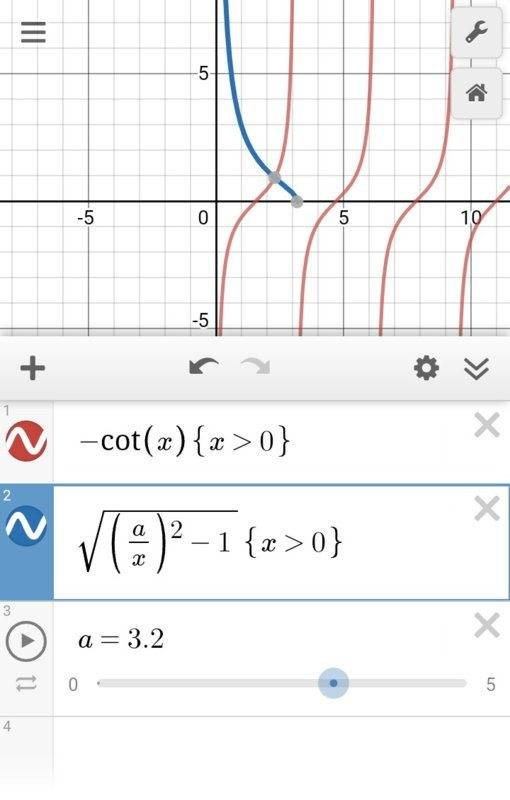# Particle in a well, can someone explain how this step works

• Theodore0101
In summary, we are trying to determine the energy for bounded states of a particle with mass m, under potential V(x) = ∞ when x<0, -V0 when 0<x<a, 0 when x>a. For part A, the relation for energy is given by tan(√(2ma^2(E+V0)/h^2)=-√(E+V0/-E). For part B, we need to find the values of V0 for which the particle has only one bounded state. This is achieved by choosing the cotangent function so that it only has one period in the corresponding interval -V0<E<0. This leads to the inequality pi^2*h^2/

#### Theodore0101

Homework Statement
A particle with mass m is effected by potential V(x) = ∞ when x<0, -V0 when 0<x<a, 0 when x>a
A) Set up a relation from which the energy for bounded states can de determined
B) For which values V0 does the particle have only one bounded state?
Relevant Equations
tan(√(2ma^2(E+V0)/h^2)=-√(E+V0/-E)
Homework Statement:: A particle with mass m is effected by potential V(x) = ∞ when x<0, -V0 when 0<x<a, 0 when x>a
A) Set up a relation from which the energy for bounded states can de determined
B) For which values V0 does the particle have only one bounded state?
Homework Equations:: tan(√(2ma^2(E+V0)/h^2)=-√(E+V0/-E)

Hi! I understand the most of this example problem, I have no problem with A), which the answer is the relation tan(√(2ma^2(E+V0)/h^2)=-√(E+V0/-E), and I mostly understand B), it is just one part in the end I can't follow. Choosing the tangens function so that it only has one period in the corresponding intervall -V0<E<0 and the equations only have one point that they meet in. Therefor we put that pi/2 < √(2ma^2(E+V0)/h^2) <3pi/2 since pi/2 to 3pi/2 is a period for a tangens function, but from there they say that the equation gives pi^2*h^2/8ma^2 < V0 < 9pi^2*h^2/8ma^2, but I don't follow this step. What happened to E, why are you allowed to take it away? Shouldn't it be pi^2*h^2/8ma^2 < V0 + E < 9pi^2*h^2/8ma^2 (instead of just V0)?

Thanks

Are you sure it is ##\tan## function only? Please check your solution. For that you don't need the restriction π/2 to 3π/2. The curve will always intersect the graph between 0 to π/2. See the graph below..

If you meant ##\cot## then the restriction applies. In that case simply note that since by defination of bound state ##-V_0 \leq E \leq 0##, ##E+V_0## is positive only if E=0 for then only inequalty is valid. See below.In both the graphs I have changed variables for my convenience.

Last edited: